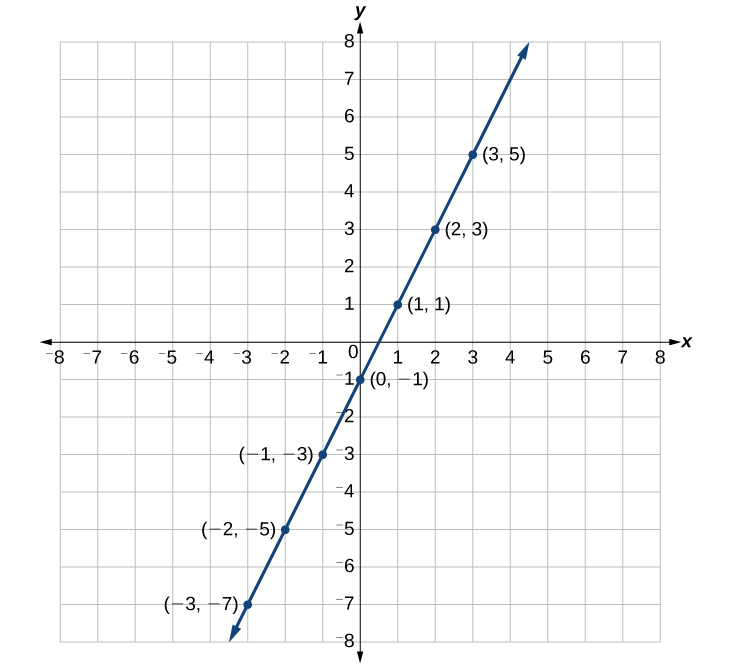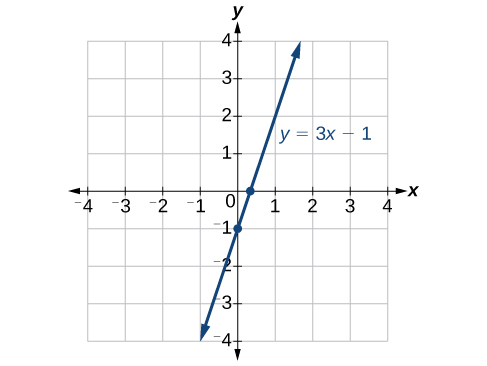## Graphing Linear Equations

### Learning Outcomes

• Plot linear equations in two variables on the coordinate plane.
• Use intercepts to plot lines.
• Use a graphing utility to graph a linear equation on a coordinate plane.

We can plot a set of points to represent an equation. When such an equation contains both an x variable and a y variable, it is called an equation in two variables. Its graph is called a graph in two variables. Any graph on a two-dimensional plane is a graph in two variables.

Suppose we want to graph the equation $y=2x - 1$. We can begin by substituting a value for x into the equation and determining the resulting value of y. Each pair of and y-values is an ordered pair that can be plotted. The table below lists values of x from –3 to 3 and the resulting values for y.

 $x$ $y=2x - 1$ $\left(x,y\right)$ $-3$ $y=2\left(-3\right)-1=-7$ $\left(-3,-7\right)$ $-2$ $y=2\left(-2\right)-1=-5$ $\left(-2,-5\right)$ $-1$ $y=2\left(-1\right)-1=-3$ $\left(-1,-3\right)$ $0$ $y=2\left(0\right)-1=-1$ $\left(0,-1\right)$ $1$ $y=2\left(1\right)-1=1$ $\left(1,1\right)$ $2$ $y=2\left(2\right)-1=3$ $\left(2,3\right)$ $3$ $y=2\left(3\right)-1=5$ $\left(3,5\right)$

We can plot these points from the table. The points for this particular equation form a line, so we can connect them. This is not true for all equations.Note that the x-values chosen are arbitrary regardless of the type of equation we are graphing. Of course, some situations may require particular values of x to be plotted in order to see a particular result. Otherwise, it is logical to choose values that can be calculated easily, and it is always a good idea to choose values that are both negative and positive. There is no rule dictating how many points to plot, although we need at least two to graph a line. Keep in mind, however, that the more points we plot, the more accurately we can sketch the graph.

### How To: Given an equation, graph by plotting points

1. Make a table with one column labeled x, a second column labeled with the equation, and a third column listing the resulting ordered pairs.
2. Enter x-values down the first column using positive and negative values. Selecting the x-values in numerical order will make graphing easier.
3. Select x-values that will yield y-values with little effort, preferably ones that can be calculated mentally.
4. Plot the ordered pairs.
5. Connect the points if they form a line.

### Example: Graphing an Equation in Two Variables by Plotting Points

Graph the equation $y=-x+2$ by plotting points.

### Try It

Construct a table and graph the equation by plotting points: $y=\frac{1}{2}x+2$.

## Using Intercepts to Plot Lines in the Coordinate Plane

The intercepts of a graph are points where the graph crosses the axes. The x-intercept is the point where the graph crosses the x-axis. At this point, the y-coordinate is zero. The y-intercept is the point where the graph crosses the y-axis. At this point, the x-coordinate is zero.

To determine the x-intercept, we set y equal to zero and solve for x. Similarly, to determine the y-intercept, we set x equal to zero and solve for y. For example, lets find the intercepts of the equation $y=3x - 1$.

To find the x-intercept, set $y=0$.

$\begin{array}{llllll}y=3x - 1\hfill & \hfill \\ 0=3x - 1\hfill & \hfill \\ 1=3x\hfill & \hfill \\ \frac{1}{3}=x\hfill & \hfill \\ \left(\frac{1}{3},0\right)\hfill & x\text{-intercept}\hfill \end{array}$

To find the y-intercept, set $x=0$.

$\begin{array}{lllll}y=3x - 1\hfill & \hfill \\ y=3\left(0\right)-1\hfill & \hfill \\ y=-1\hfill & \hfill \\ \left(0,-1\right)\hfill & y\text{-intercept}\hfill \end{array}$

We can confirm that our results make sense by observing a graph of the equation. Notice that the graph crosses the axes where we predicted it would.### How To: Given an equation, find the intercepts

1. Find the x-intercept by setting $y=0$ and solving for $x$.
2. Find the y-intercept by setting $x=0$ and solving for $y$.

### Example: Finding the Intercepts of the Given Equation

Find the intercepts of the equation $y=-3x - 4$. Then sketch the graph using only the intercepts.

### Try It

Find the intercepts of the equation and sketch the graph: $y=-\frac{3}{4}x+3$.

## Using a Graphing Utility to Plot Lines

You can use an online graphing tool to quickly plot lines. Watch this short video Tutorial to learn how.

### Try It

Desmos has a helpful feature that allows you to turn a constant (number) into a variable. Follow these steps to learn how:

1. Graph the line $y=-\frac{2}{3}x-\frac{4}{3}$.
2. On the next line enter $y=-a x-\frac{4}{3}$. You will see a button pop up that says “add slider: a”, click on the button. You will see the next line populated with the variable a and the interval on which a can take values.
3. What part of a line does the variable a represent? The slope or the y-intercept?